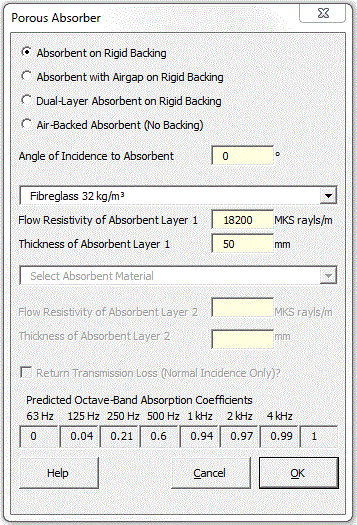### Strutt Help

Absorption or Trasmission Coefficients of Porous Absorber    1/1,1/3

Strutt|Building Acoustics|Porous Absorber calculates the absorption coefficients or transmission loss of a porous absorber, and inserts the calculated values into the active row of the worksheet.

Strutt calculates absorption coefficients for the following absorber geometries:

• Porous absorber on rigid backing
• Porous absorber with airgap on rigid backing
• Dual-layer porous absorber on rigid backing
• Air-backed porous absorber (i.e. no backing)
For the air-backed absorber geometry, Strutt also allows the calculation of the transmission loss through the porous material.Strutt calculates the absorption coefficients alpha from the complex pressure-reflection factor R between the surface of the absorber and the air using the following formula:

R = (Z_S cos(phi)-Z_0)/(Z_S cos(phi)+Z_0)

alpha = 1-|R|^2

where:
Z_0 is the characteristic impedance (rho C) of air
Z_S is the surface impedance of the absorber
phi is the angle of incidence of the incident sound field

Strutt calculates the normal-incidence transmission loss using the complex pressure-transmission factor T of the construction and the power transmission coefficient tau using the following formulae:

T = (2Z_S)/(Z_S+Z_0)

tau = |T|^2

"TL" = 10log_"10"(tau)

The surface impedance of one layer of the absorber (Z_(Si+1)) is related to the surface impedance of the layer below it (Z_(Si)) using:

Z_(Si+1) = (-j Z_(Si) Z_i cot(k_(x i) d_i) + Z_i^2)/(Z_(Si) - j Z_i cot(k_(x i) d_i))

where:
d_i is the thickness of the layer
Z_i is the characteristic impedance of the layer
k_(x i) is the wavenumber for normal-incidence sound propagation in the layer

The surface impedance of the bottom layer of the absorber is found using the above formula, but imposing either:

• a boundary condition where the lower surface is rigid (and therefore has infinite impedance). In this case the formula reduces to:

Z_1 = -j Z_i cot(k_(x i) d_i)
• a boundary condition where the absorber is backed by a semi-infinite airspace, in which case:

Z_(Si)=Z_0

For air, Z_i = Z_0 and k_(x i) = k_0 = omega//c

For porous absorbent, Z_i and k_(x i) are calculated using the Delaney and Bazley formulation:

Z_i = rho_0 c_0 (1 + 0.0571X^(-0.754) - j 0.087X^(-0.732))

k_i = k_0 (1 + 0.0978X^(-0.7)-j0.189X^(-0.595))

where X is a frequency-dependent parameter given by:

X = (rho_0 f)/sigma

sigma is the flow resistivity (MKS rayls/m) of the porous absorbent.

A drop-down menu is provided giving flow-resistivity values for several common absorbent materials, or else the user may enter in the flow resistivity manually.

For oblique-incidence, the underlying theory is the same, except the complex wavenumber k_i now includes both normal k_(x i) and lateral k_(y i) propagation components:

Z_(Si+1) = (-j Z_(Si) Z_i k_i/k_(x i) cot(k_(x i) d_i) + Z_i^2)/(Z_(Si) - j Z_i k_i/k_(x i) cot(k_(x i) d_i))

k_(x i) = sqrt(k_i^2-k_(i+1)^2 sin(phi_(i+1)))

For multi-layer absorbers, the incidence angle phi_i in each absorber will vary according to Snell's Law and the relative speed of sound (and hence impedance/wave number) in each layer

Reference: TJ Cox and P D'Antonio (2004) Acoustic Absorbers and Diffusers: Theory, Design and Application, pp141-150 Spon Press